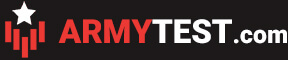Select Page

# ADF YOU Session Question Categories

The Job Opportunities Assessment (JOA) consists of 51 test questions with a time limit of 20 minutes.

The majority of the questions are multiple-choice questions with only one or two correct answers. The question will state whether you are expected to give one or two answers to the question.

The most common question categories of the JOA are:

### Word Problems

Contain many different types of mathematical word problems.

### Number Series

You are required to find the missing number in a series of numbers. The logic behind the questions is mostly mathematical.

#### Number Rows

You must find the missing number in a simple table containing two rows and six columns.

Word Code

You are given a coded message as well as the same message decoded. You are then given another coded message and must decode it.

Deductive Reasoning

Deductive reasoning questions will require you to use your problem-solving and reasoning skills to evaluate arguments and draw logical conclusions.
For deductive reasoning questions, you must draw conclusions based only on the information given in the question and not your own knowledge. If the conclusion cannot be drawn from the information given, then the conclusion does not follow.
You will be presented with a list of (usually 5) statements. You are required to find the two statements that together will allow you to reach a certain conclusion.

### Word Meanings

Word Meaning questions require that you find the connection between a list of words.

### Word Analogy

In the Word Analogy questions the reader is to hypothesize the relationship between the two example words and then find the best match between the option words.

### Abstract Reasoning

Find the figure that complete the series of figures.

#### Odd one out

You are shown 5 or 6 shapes/items and you are required to identify the rules and similarities between them, in order to find the correct answer. You will be asked to find either one or two items that does not belong with the rest.

All the question categories described above are included in our test prepartion software. In the software you will be introduced to each type of question one at a time.

Want samples of the question categories? Consider taking our free ADF sample test to try these questions yourself.